© | Dror Bar-Natan: Publications: WKO: < >

# wClips-120314

#### Speaker: Karene Chu

 width: 400 720 orig/wClips-120314.mpg For now, this video can only be viewed with web browsers that support

Notes on wClips-120314:    [edit, refresh]

Section 3.6 - the relation with Lie algebras.

Announcements. small circle, UofT, LDT Blog (also here). Email Dror to join our mailing list!

Resources. How to use this site, Dror's notebook, blackboard shots.

### The wClips

http://katlas.math.toronto.edu/drorbn/dbnvp/dbnvp.png
Jan 11, 2012 : Introduction.
: Section 2.1 - v-Braids.
Jan 18, 2012 : An introduction to this web site.
: Section 2.2 - w-Braids by generators and relations and as flying rings.
: Section 2.2 - w-Braids - other drawing conventions, "wens".
Jan 25, 2012 : Section 2.2.3 - basis conjugating automorphisms of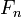$F_n$.
: A very quick introduction to finite type invariants in the "u" case.
Feb 1, 2012 : Section 2.3 - finite type invariants of v- and w-braids, arrow diagrams, 6T, TC and 4T relations, expansions / universal finite type invariants.
Feb 8, 2012 : Review of u,v, and w braids and of Section 2.3.
Feb 15, 2012 : Section 2.5 - mostly compatibilities of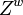$Z^w$, also injectivity and uniqueness of$Z^w$.
Feb 22, 2012 : Section 2.5.5,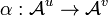$\alpha:{\mathcal A}^u\to{\mathcal A}^v$, and Section 3.1 (partially), the definition of v- and w-knots.
Feb 29, 2012 : Sections 3.1-3.4: v-Knots and w-Knots: Definitions, framings, finite type invariants, dimensions, and the expansion in the w case.
Mar 7, 2012 : Section 3.5: Jacobi diagrams and the bracket-rise theorem.
Mar 14, 2012 : Section 3.6 - the relation with Lie algebras.
Mar 21, 2012 : Section 4 - Algebraic Structures.
Mar 28, 2012 Out-of-sequence not-on-tape we watched the video of Talks: GWU-1203.
Apr 4, 2012 : Section 3.7 - The Alexander Theorem (statement).
Apr 18, 2012 : Aside on the Euler trick, the differential of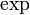$\exp$, and the BCH formula.
Apr 25, 2012 : Section 3.8, a disorganized lecture towards the proof of the Alexander theorem.
May 2, 2012 : Section 4: Algebraic structures (review), circuit algebras, v- and w-tangles.
May 10, 2012 : Sections 5.1 and 5.2: tangles, their projectivization and its relationship with Alekseev-Torossian spaces.
May 23, 2012 : Section 5.2: Proof of the relationship with A-T spaces.
May 30, 2012 : Interpreting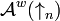${\mathcal A}^w(\uparrow_n)$ as a universal space of invariant tangential differential operators.
Group photo on January 11, 2012: DBN, ZD, Stephen Morgan, Lucy Zhang, Iva Halacheva, David Li-Bland, Sam Selmani, Oleg Chterental, Peter Lee.
refresh
panel
Managed by dbnvp: Click "Comment on h:mm:ss" below the video to add a comment on a specific time.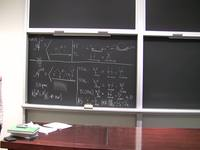0:00:00  Blackboard shots by Zsuzsanna Dancso.

Sorry, for this wClip we've used a different video camera than the usual (the usual one was in ), and there are several bugs in this page as a result. --Drorbn 21:45, 20 March 2012 (EDT)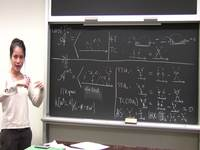0:04:47  No need to forget about the algebra structure - this is an algebra isomorphism, and even a bialgebra isomorphism. --Drorbn 18:38, 19 March 2012 (EDT)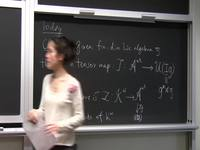0:11:34  It is great to know the relationship with Lie bialgebras, as it is extremely relevant for the study of ${\mathcal A}^v$. Yet it is also good to know that $I{\mathfrak g}$ has a much simpler definition, that avoids some of the complexity. Namely, $I{\mathfrak g}$ is the semi-direct product ${\mathfrak g}^\star\rtimes{\mathfrak g}$, where ${\mathfrak g}$ acts on its dual ${\mathfrak g}^\star$ using the coadjoint action. The metric on $I{\mathfrak g}$ need not ever be explicitly used, yet it is the metric associated with the a norm on $I{\mathfrak g}$, which is simply the contraction map of ${\mathfrak g}^\star$ and ${\mathfrak g}$. This definition appears roughly starting at minute 44:00 of this video. --Drorbn 20:30, 19 March 2012 (EDT)